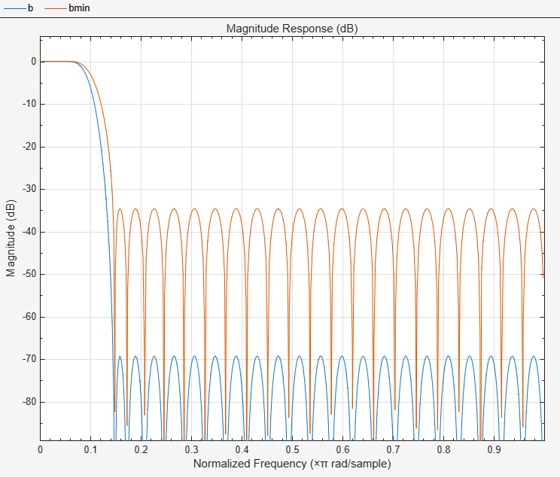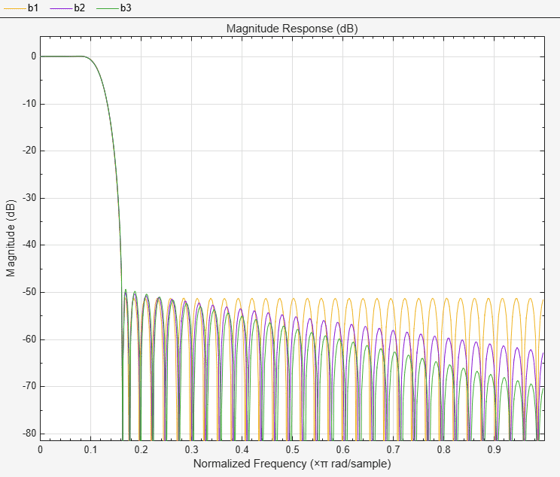firnyquist

Lowpass Nyquist (Lth-band) FIR filter

Syntax

b = firnyquist(n,l,r)
b = firnyquist('minorder',l,r,dev)
b = firnyquist(n,l,r,decay)
b = firnyquist(n,l,r,'nonnegative')
b = firnyquist(n,l,r,'minphase')

Description

b = firnyquist(n,l,r) designs an Nth order, Lth band, Nyquist FIR filter with a rolloff factor r and an equiripple characteristic.

The rolloff factor r is related to the normalized transition width tw by tw = 2π(r/l) (rad/sample). The order, n, must be even. l must be an integer greater than one. If l is not specified, it defaults to 4. r must satisfy 0< r < 1. If r is not specified, it defaults to 0.5.

b = firnyquist('minorder',l,r,dev) designs a minimum-order, Lth band Nyquist FIR filter with a rolloff factor r using the Kaiser window. The peak ripple is constrained by the scalar dev.

b = firnyquist(n,l,r,decay) designs an Nth order (n), Lth band (l), Nyquist FIR filter where the scalar decay, specifies the rate of decay in the stopband. decay must be nonnegative. If you omit or leave it empty, decay defaults to 0 which yields an equiripple stopband. A nonequiripple stopband (decay ≠0) may be desirable for decimation purposes.

b = firnyquist(n,l,r,'nonnegative') returns an FIR filter with nonnegative zero-phase response. This filter can be spectrally factored into minimum-phase and maximum-phase “square-root” filters. This allows you to use the spectral factors in applications such as matched-filtering.

b = firnyquist(n,l,r,'minphase') returns the minimum-phase spectral factor bmin of order n. bmin meets the condition b=conv(bmin,bmax) so that b is an Lth band FIR Nyquist filter of order 2n with filter rolloff factor r. Obtain bmax, the maximum phase spectral factor by reversing the coefficients of bmin. For example, bmax = bmin(end:-1:1).

Examples

collapse all

This example designs a minimum phase factor of a Nyquist filter.

bmin = firnyquist(47,10,.45,'minphase');
b = firnyquist(2*47,10,.45,'nonnegative');
[h,w,s] = freqz(b); hmin = freqz(bmin);
fvtool(b,1,bmin,1);This example compares filters with different decay rates.

b1 = firnyquist(72,8,.3,0); % Equiripple
b2 = firnyquist(72,8,.3,15);
b3 = firnyquist(72,8,.3,25);
fvtool(b1,1,b2,1,b3,1);References

T. Saramaki, Finite Impulse Response Filter Design, Handbook for Digital Signal Processing, Mitra, S.K. and J.F. Kaiser Eds. Wiley-Interscience, N.Y., 1993, Chapter 4.

Extended Capabilities